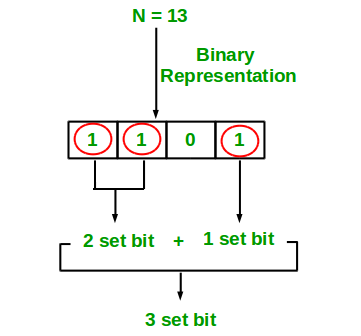# Python Program to Count set bits in an integer

• Difficulty Level : Basic
• Last Updated : 02 Jan, 2019

Write an efficient program to count number of 1s in binary representation of an integer.

Examples :

```Input : n = 6
Output : 2
Binary representation of 6 is 110 and has 2 set bits

Input : n = 13
Output : 3
Binary representation of 11 is 1101 and has 3 set bits
```## Recommended: Please solve it on “PRACTICE ” first, before moving on to the solution.

1. Simple Method Loop through all bits in an integer, check if a bit is set and if it is then increment the set bit count. See below program.

## Python3

 `# Function to get no of set bits in binary``# representation of positive integer n */``def`  `countSetBits(n):``    ``count ``=` `0``    ``while` `(n):``        ``count ``+``=` `n & ``1``        ``n >>``=` `1``    ``return` `count`` ` ` ` `# Program to test function countSetBits */``i ``=` `9``print``(countSetBits(i))`` ` `# This code is contributed by``# Smitha Dinesh Semwal`
Output:

```2
```

Recursive Approach :

## Python3

 `# Python3 implementation of recursive``# approach to find the number of set``# bits in binary representation of ``# positive integer n`` ` `def` `countSetBits( n):``     ` `    ``# base case``    ``if` `(n ``=``=` `0``):``        ``return` `0`` ` `    ``else``:`` ` `        ``# if last bit set add 1 else``        ``# add 0``        ``return` `(n & ``1``) ``+` `countSetBits(n >> ``1``)``         ` `# Get value from user``n ``=` `9`` ` `# Function calling``print``( countSetBits(n))     ``         ` `# This code is contributed by sunnysingh`
Output:
```2
```

Please refer complete article on Count set bits in an integer for more details!

My Personal Notes arrow_drop_up